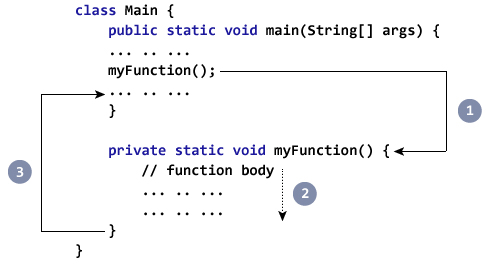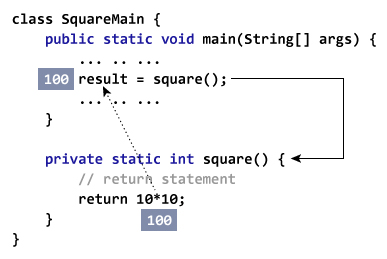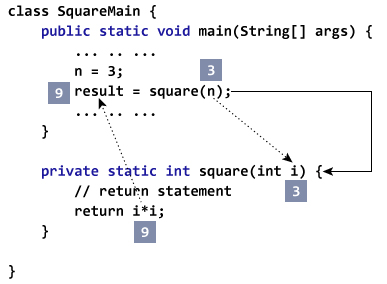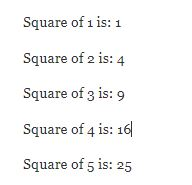# blog posts# In this tutorial, you will learn about Java methods, how to define a method and use them in the program with the help of an example.

## What is a method?

In mathematics, you read about functions. For example, f (x) = x 2 is a function that returns the square value of x.

If x = 2, then f (2) = 4

If x = 3, f (3) = 9

and so on.

Similarly, in programming, it is a block function of code that performs a specific task.

In object-oriented programming, a method is a term used for a function. Methods are limited to one class and define the behavior of a class.

## Types of Java methods

The method is of two types, depending on whether it is user-defined or available in the standard library:

• Standard library methods
• User-defined methods

### Standard library methods

Standard library methods are internal methods in Java that are easy to use. These standard libraries are provided with the Java Class Library (JCL) in the Java Archive (* .jar) with JVM and JRE.

For example,

• print () is a method from java.io.PrintSteam. (“…”) Print prints the string inside the quotation marks.
• sqrt () is a method for the Math class. Root returns the number.

Consider the following example:

1. public class Numbers {
2. public static void main (String [] args) {
3. System.out.print (“Square root of 4 is:” + Math.sqrt (4));
4. }
5. }

Output

Square root of 4 is: 2.0

### User-defined method

You can also define methods within the class as you wish. These methods are called user-defined methods.

How to create a user-defined method?

You must define it before using it (using the method).

Here’s how to define methods in Java.

public static void myMethod () {

System.out.println (“My Function called”);

}

Here is a method called myMethod () defined.

#### Before the function name, you can see the three keywords public, static and void.

• The public keyword popularizes the myMethod () method. Public members can be accessed from outside the classroom.
• The static keyword indicates that the method can be accessed without creating an object of the class.
• The void keyword indicates that this method does not return any values.

In the above program, our method does not accept any arguments. Hence the parentheses are empty ().

Structure of defining a Java method:

modifier static returnType nameOfMethod (Parameter List) {

// method body

}

#### Here,

• modifier – Defines the type of access whether this method is public, private, etc.
• static – If you use the static keyword in the method, it becomes a static method. Static methods can be called from the class without creating an object.

For example, the sqrt () method of the standard Math class is static. Therefore, we can use Math.sqrt directly without creating an object of class Math.

• ReturnType – A method can return a value.

Which can return local data types (int, float, double, etc.), local objects (string, map, list, etc.) or any internal and user-defined object.

If the method does not return a value, its return type is void.

• nameOfMethod – The method name is an identifier.

You can give the method any name. However, it is more common to name it based on the tasks it performs. For example, calcuteInterest, calcuteArea and so on.

• Parameters (arguments – Parameters are values ​​passed to the method. You can pass any number of arguments to the method.
• Metho body – Specifies what the method actually does, how parameters are manipulated by programming commands, and what values ​​are returned. The code inside {} is the body of the method.

#### How to call the Java method?

Now that you have defined the method, you must use it. To do this, you must call the method:

myMethod ();

This command invokes the myMethod () method that was previously defined.1. While Java executes the program code, it encounters myMethod (). (In code)

2. The execution then goes to the myFunction () method and executes the code inside the body of the method.

3- After executing the code inside the body of the method, the program returns to the main state and executes the next expression.

Example: Java method program

By defining a Java class, let’s look at a Java method in action.

1. class Main {
2. public static void main (String [] args) {
3. System.out.println (“About to encounter a method.”);
4. // method call
5. myMethod ();
6. System.out.println (“Method was executed successfully!”);
7. }
8. // method definition
9. private static void myMethod () {
10. System.out.println (“Printing from inside myMethod ()!”);
11. }
12. }

#### When you run the program, the output will be equal to:

Printing from inside myMethod ().

Method was executed successfully!

The myMethod () method in the above program does not accept any arguments. Also, this method does not return any values ​​(the return type is void).

Note that, we have named this method without creating a class object. This was possible because myMethod () is static.

#### In the example below, our method is non-static and is inside another class.

1. class Main {
2. public static void main (String [] args) {
3. Output obj = new Output ();
4. System.out.println (“About to encounter a method.”);
5. // calling myMethod () of Output class
6. obj.myMethod ();
7. System.out.println (“Method was executed successfully!”);
8. }
9. }
10. class Output {
11. // public: this method can be called from outside the class
12. public void myMethod () {
13. System.out.println (“Printing from inside myMethod ().”);
14. }
15. }

#### When you run the program, the output will be equal to:

Printing from inside myMethod ().

Method was executed successfully!

Note that we first created an object of class Output. Because myMethod () is a non-static method.

### Java methods with received arguments and return values

A Java method can have zero or more received arguments and may return a value.

Example: The return value of a method

Let’s give an example and return some value from the method.

1. class SquareMain {
2. public static void main (String [] args) {
3. int result;
4. result = square ();
5. System.out.println (“Squared value of 10 is:” + result);
6. }
7. public static int square () {
8. // return statement
9. return 10 * 10;
10. }
11. }

#### Output

Squared value of 10 is: 100

In the above code snippet, the squre () method does not accept any arguments and always returns a square value of 10.

Note that the recursive type (squre) is an integer. This means that the method returns the value of an integer.As you can see, the scope of this method is limited because it always returns the same value.

Now let’s modify the above code snippet so that instead of always returning the square value of 10, it returns the square value of each integer that is passed to the method.

#### Example: Method with received arguments and return value

1. public class SquareMain {
2. public static void main (String [] args) {
3. int result, n;
4. n = 3
5. result = square (n);
6. System.out.println (“Square of 3 is:” + result);
7. n = 4
8. result = square (n);
9. System.out.println (“Square of 4 is:” + result);
10. }
11. static int square (int i) {
12. return i * i;
13. }
14. }

#### Output

Squared value of 3 is: 9

Squared value of 4 is: 16

Now, the squre () method returns the square value of each integer passed to it.Java is a completely dependent language. If you use any data type other than int (in the example above), the compiler will get an error.

The n argument in the getSquare () method is called a real argument when calling a method.

result = getSquare (n);

The i-parameter accepts the arguments passed in the getSquare (int i) method definition, which is called the formal argument. The type of the formal argument must be explicitly typed.

#### You can use a comma to send more than one argument to a Java method. For example,

1. public class ArithematicMain {
2. public static int getIntegerSum (int i, int j) {
3. return i + j;
4. }
5. public static int multiplyInteger (int x, int y) {
6. return x * y;
7. }
8. public static void main (String [] args) {
9. System.out.println (“10 + 20 =” + getIntegerSum (10, 20));
10. System.out.println (“20 x 40 =” + multiplyInteger (20, 40));
11. }
12. }

#### Output

10 + 20 = 30

20 x 40 = 800

The data type of the real and formal arguments must match, ie the data type of the first real argument must match the first formal argument. Likewise, the type of the second real argument must match the type of the second formal argument, and so on.

Example: Calculate the squares of the numbers 1 to 5

1. public class JMethods {
2. // method defined
3. private static int getSquare (int x) {
4. return x * x;
5. }
6. public static void main (String [] args) {
7. for (int i = 1; i <= 5; i ++) {
8. // method call
9. result = getSquare (i)
10. System.out.println (“Square of” + i + ”is:” + result); }
11. }
12. }

#### OutputIn the above code snippet, the getSquare () method considers int as a parameter. Based on the argument submitted, the method returns its square value.

Here, the i argument of type int is passed to the getSquare () method during the method call.

result = getSquare (i);

The x parameter accepts the submitted argument. [In function definition (getSquare (int x)]

return i * i; : Is the return command and returns the value by calling method and terminates the function.

Did you notice we used the getSquare method 5 times?

## What are the advantages of using methods?

• The main advantage is the ability to reuse the code. You can write a method once, and use it several times. You do not have to rewrite the entire code each time. Think about “write once, reuse several times.”
• Methods make code more readable. For example, the getSalaryInformation () method is more readable than reading lines of code so we can know what this method will do.